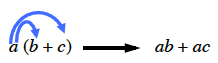### Home > CC2MN > Chapter 5 > Lesson 5.2.4 > Problem5-70

5-70.

Use the Distributive Property to simplify the following expressions.

The Distributive Property involves multiplying the number outside the parentheses into each individual value inside the parentheses and then combining the terms.1. $4(x+2)$

1. $-5(9+x)$

$-45-5x$

Be sure you know how to get this answer.

1. $7(x-3)$